# Context modules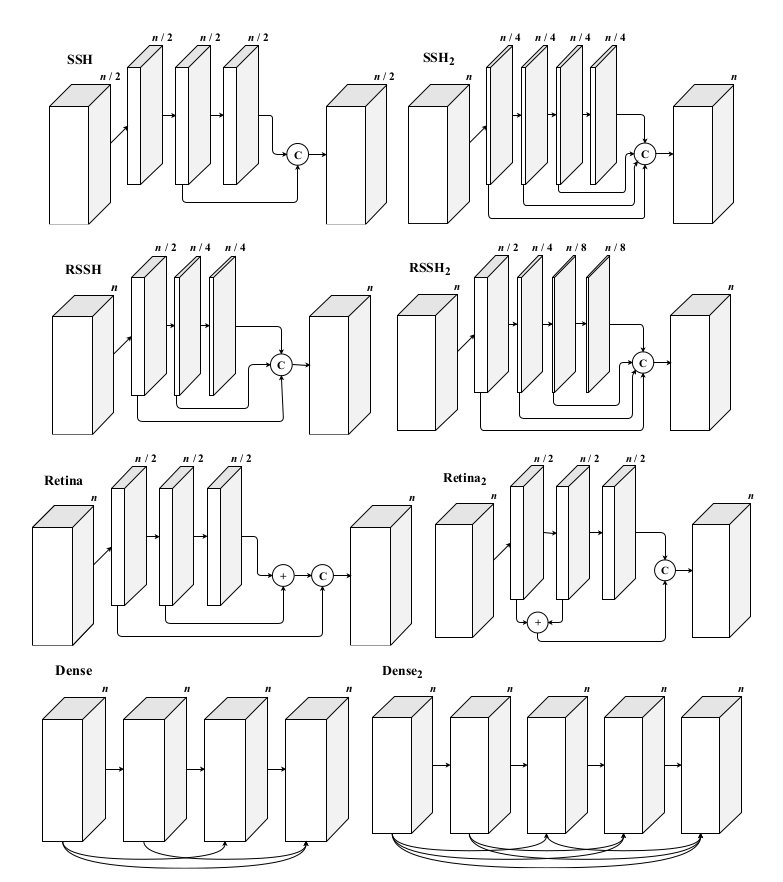Fig.1: Illustration of the context modules used by Earp et al. , where n denotes the number of filters in the first 3x3 convolution layer, C, denotes concatenation, and + denotes vector addition. The left column are common context modules: SSH context module is from , both the RSSH and Retina modules are from , and the Dense context module is from . The right column are slight permutations of these modules. The number of filters, n, in the context module is different for each network backbone. This figure corresponds to Figure 1 from the paper .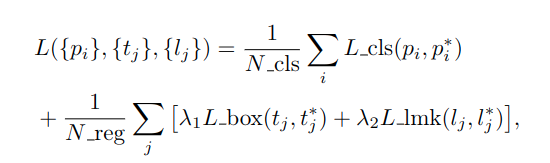Eq. 1: Multi-task loss function where λ1,2 are scale factors which are set to 0.25 and 0.1, i and j correspond to all and positive anchors, N_cls and N_reg are the batch size and number of anchors, respectively.

# Models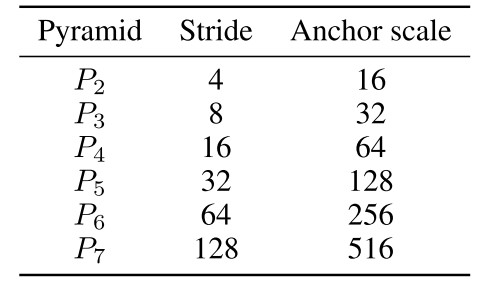Table 1: The pyramid setup Earp et al.  use for all of the experiments. The stride denotes the factor by which the original image have been scaled by, and the scale is the factor that is applied to the anchor size.

# Results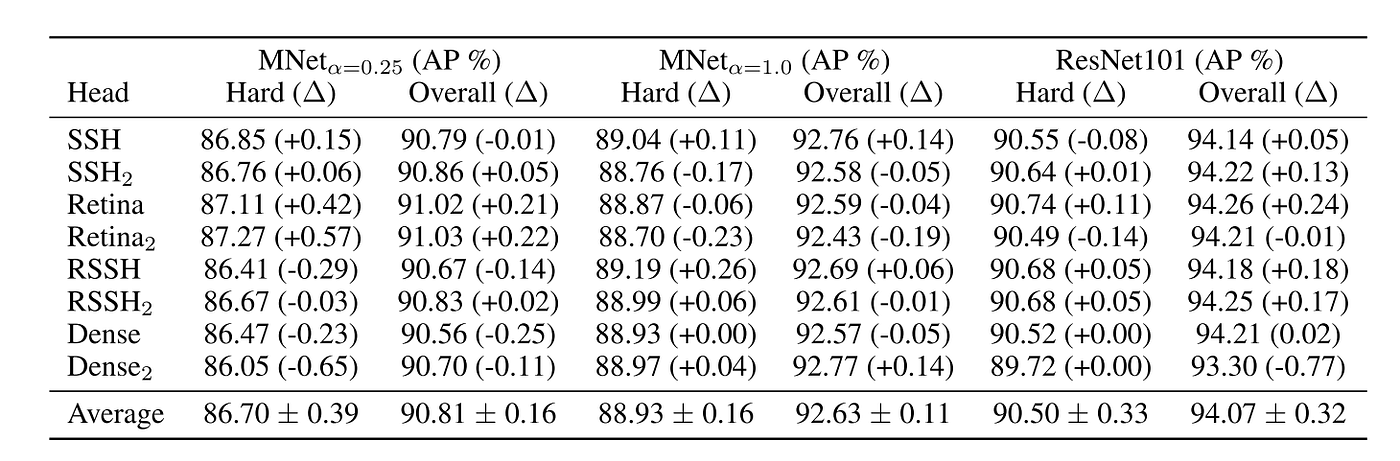Table 2: The WIDER FACE validation average precision (AP) for each backbone and each context module. The value in parentheses denotes the APs divergence (∆) from the mean. The ‘Hard’ columns show the APs on the ‘hard’ set, and the ‘Overall’ columns show the mean APs across all three sets. The bottom row shows the mean APs and standard deviation for all context modules.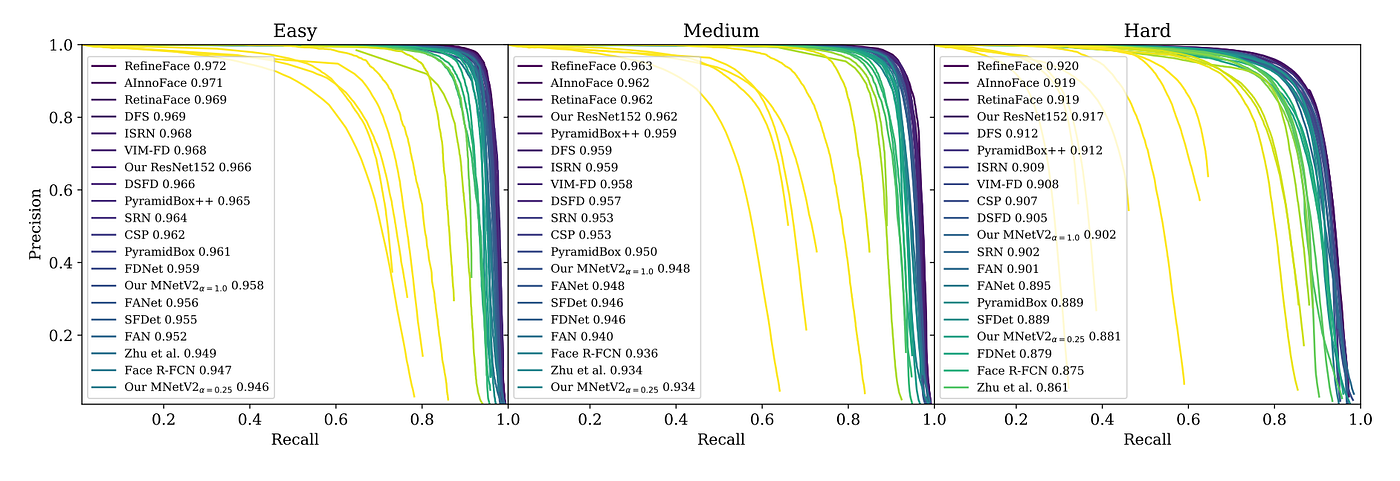Fig. 2: Final results for the face detection models developed by Earp et al.  on the WIDER FACE validation set, evaluated in 2019. The figure shows the curves for the top 20 submissions on each set. This figure corresponds to Figure 2 from the paper .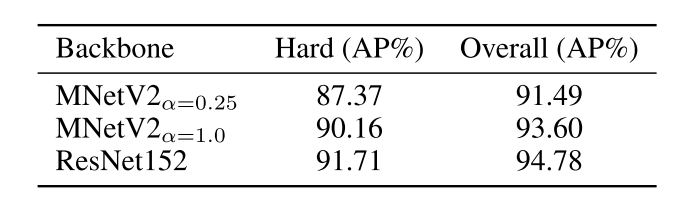Table 3: Average precision of each backbone on the WIDER FACE Hard set and averaged over all sets.

--

--